# Moments

## Introduction

Try pushing a door with your hand on the middle of the door. Then try pushing the same door with your hand on the handle at the far end of the door.

The second time was easier right? That's because you applied more moment force about the door hinge. Moment force, or torque, is the tendency of a force to rotate an object about an axis.

Specifically, if we apply a force $F$ about some pivot point, then

$$M = Fd$$

Where $M$ is the moment force in Nm (Newton metres) due to the force $F$ in N and $d$ is the shortest distance to the line of action of the force in metres.

### Example

Q) Find the moment force about the pivot point $P$ due to the 5N force in the diagram below.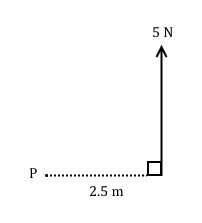A) We have conveniently been given the shortest distance to the line of action of the 5N force. So

$$M = 5 \times 2.5 = 12.5 \textrm{Nm}$$

### Example

Q) Find the moment force about the pivot point $P$ due to the 10N force in the diagram below.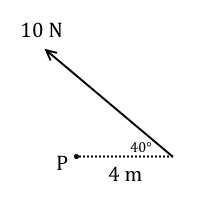A) We know the force but we need to know the shortest distance to the line of action of the force.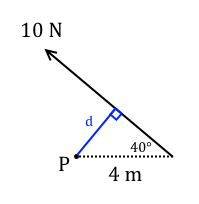$$d = 4\sin(40) = 2.57 \textrm{m} \left(\textrm{3.s.f.}\right)$$

$$\therefore M = 10 \times 2.57 = 25.7 \textrm{Nm}\left(\textrm{3.s.f.}\right)$$

## Equilibrium of a rigid body

### Resolving moments

For there to be equilbrium about a pivot point we need the sum of the moments acting around it to be zero.

We resolve moments about a pivot point just like we resolve forces. The convention is anti-clockwise positive, meaning moments acting anti-clockwise are taken as positive and clockwise moments are negative.

When we resolve moments about a pivot point we take moments about that point, ignoring any forces that act directly through the point itself.

### Example

Q) Is the following system in rotational equilibrium? Find the resultant of all the moment forces about pivot point $P$.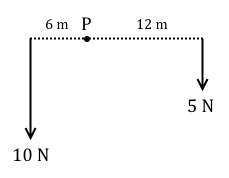A) Yes because $\left(10 \times 6\right) - \left(5 \times 12\right) = 0$.

### Bringing it all together

In M1 exam questions you will need to find the equilibrium conditions of a rigid body. That means you need to make sure the body is in static equilbrium and rotational equilbrium, which can be thought of as horizontal or static equilibrium for most cases in M1.

That means you need the sum of the forces acting in two dimension to equal zero, and the sum of the moments about all the relevant points to equal zero.

$$\sum F_x = 0$$

$$\sum F_y = 0$$

$$\sum M = 0$$

You will need to take certain extra things into account. If a rigid body sits on supports, then Newton's third law states that each support will exert a reaction force back upwards perpendicular to the length of the body.

Additionally, if you are told the mass of the body itself, you will need to take that into account. In M1 the body is almost always modelled as a horizontal straight line, so it suffices to draw the weight of the body vertically downwards through the centre of the body.

### Example

Q) A 10kg beam of length 3m sits on two supports at either of its ends. Find the reaction forces from both of the supports.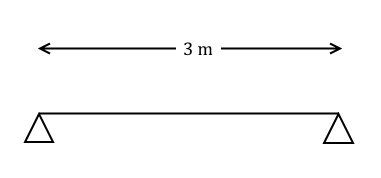A) We're told that the beam has mass 10kg, so its weight is $10g \textrm{N}$, which we model as going vertically downwards through the centre of the beam. Label the left and right supports $A$ and $B$ respectively, and draw on the reaction forces at either support too.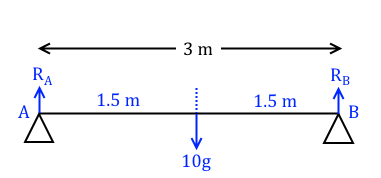There are no horizontal forces so we only need look at vertical equilibrium of forces.

$$R_A + R_B = 10g$$

We also need to take moments about one of the supports to ensure rotational equilibrium. Let's pick $A$.

$$\left(R_B \times 3\right) - \left(10g\times 1.5\right) = 0$$

$$\therefore R_B = \frac{10g \times 1.5}{3} = 5g = 49 \textrm{N}$$

From vertical equilibrium we know that $R_A + R_B = 10g$ so we can find $R_A$

$$R_A = 10g - 5g = 5g = 49 \textrm{N}$$

### Example

Q) A 5kg rod of length 10m is held in horizontal equilibrium by a cable at end $A$ and a support at end $B$. A particle of mass 5kg is placed $x$ metres away from end $A$. Given that the tension in the cable at $A$ is twice the magnitude of the reaction at support $B$, find the value of $x$.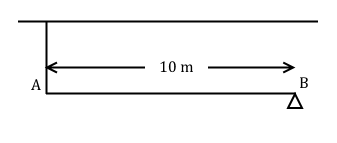A) The rod has mass 5kg so its weight is $5g \textrm{N}$, which acts through the centre of the rod. The weight of the particle is also $5g \textrm{N}$. Label the tension in the cable as $T$ and the reaction at $B$ as $R$. Draw on all of the forces.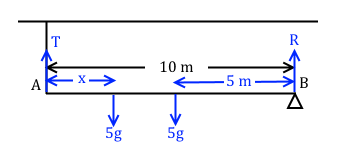Taking vertical equilibrium of forces

$$T + R - 5g - 5g = 0$$

$$\therefore T+R = 10g$$

We were told that $T = 2R$ so

$$3R = 10g \Rightarrow R = \frac{10}{3}g \textrm{ and } T = \frac{20}{3}g$$

Now take moments about point $A$

$$10R - 5gx - \left(5g \times 5\right) = 0$$

Subbing in $R = \frac{10}{3}g$ and solving for $x$

$$\left(10\times \frac{10}{3}g\right) - 5gx - 25g = 0$$

$$5x = \frac{100}{3} - 25$$

$$\therefore x = \frac{5}{3} = 1.67 \textrm{m} \left(\textrm{3.s.f.}\right)$$